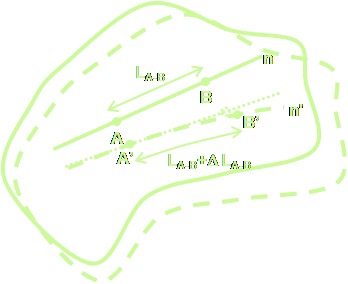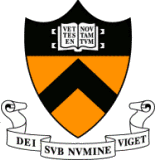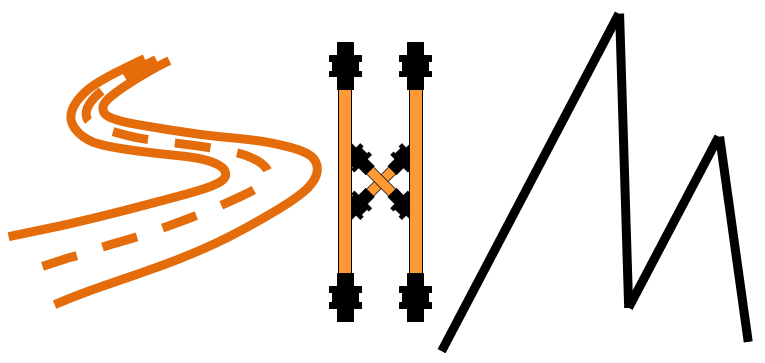BRANKO GLISICHOME
BACKCourse CEE 521: Continuum mechanics INTRODUCTION This course deals with advanced theory regarding stress, strain, and deformation or flow in solids, liquids, and gases. The course focuses to materials that can be considered as continuous, with no gaps, pores, or other empty spaces (molecular structure is disregarded). Stress at a point of material (or media) is defined as mathematical limit, similar to as the derivative is defined at a point in differential calculus. Distributions of stress in material (or media) are then studied using common calculus methods. Consequently, physical models that can be easily visualized and understood are built and presented. The theory gives a very good approximation of reality; quantitative predictions agree with experimental results and are applied in theories of elasticity, plasticity, and fluid mechanics under wide range of conditions. 80-minute lectures are held twice a week. Although strong background in linear algebra, calculus and engineering mechanics is required, students with weaker backgrounds will be able to follow the course to a satisfactory extent. OBJECTIVES Student taking the course will: Enhance knowledge in higher level mathematics and adopt mathematical language necessary for learning the theory and conducting the research related to mechanics of materials; Learn the theory describing mechanics of continuous media including both solids and fluids, and understand associated physical principles; Create solid basis for the research requiring higher level knowledge in mechanics, such as numerical modeling with finite element methods, and beyond. GRADING A-F, P, Aud Class/precept participation (homework) 30%, midterm exam 25%, final exam 45% Ask-professor sessions (voluntarily) COURSE SCHEDULE Lectures: Tuesdays and Thursdays, 9:30AM - 10:50AM Room: Friend 110 SAMPLE READING LIST 1) Lawrence E. Malvern, Introduction to the Mechanics of a Continuous Medium, Prentice-Hall, Inc., 1977 2) A. Cemal Eringen: Mechanics of Continua, John Wiley & Sons, Inc., 1967 3) George E. Mase and G. Thomas Mase: Continuum mechanics for engineers, CRC Press, Inc., 1992 DRAFT OUTLINE Introduction to the continuum mechanics. Main hypothesis of the continuous medium. Vectors and tensors. Vectors; Vector and scalar components; Indicial notation; Scalar product and vector product of vectors; Change of orhtonormal basis; Tensors; Rectangular Cartesian tensor components; Dyadics; Tensor properties; Vector and tensor calculus; Differentiation, gradient, divergence and curl. Stress. Body forces and surface forces. Traction or stress vector. Stress components. Principal axes of stress and principal stresses. Invariants. Spherical and deviatoric stress tensors; Mohr’s circles; Plane stress; Stress resultants in the simplified theory of bending of thin plates. Strain and deformation. Small strain and rotation in two and three dimensions; Kinematics of a continuous medium; Rate-of-deformation tensor; Spin tensor; Natural strain increment; Finite strain and deformation; Geometric measures of strain; Relative deformation gradient; Rotation and stretch tensors; Compatibility conditions; Determination of displacements. General principles. Introduction; Integral transformations; Flux; Conservation of mass; The continuity equation. Momentum principles; Equations of motion and equilibrium; Couple stresses; Energy balance; First law of thermodynamics; Energy equation; Principle of virtual displacements; Entropy and the second law of thermodynamics; The caloric equation of state. Constitutive equations. Introduction; Ideal materials; Classical elasticity; Generalized Hook’s law; Isotropy; Hyperelasticity; The strain energy function; Elastic symmetry; Thermal stresses; Fluids; Ideal fluid; Linearly viscous fluid; Stokes condition; Laminar and turbulent flow; Linear viscoelastic response; Plasticity; Plastic behavior of materials; Examples of theories; Perfectly plastic materials; Visco-plastic materials. Fluid mechanics. Field equations of Newtonian fluid: Navier-Stokes equations. Perfect fluid: Euler equation. Kelvin’s theorem. Bernoulli equation. Irrotational flow. Velocity potential. Acoustic waves. Gas dynamics. Potential flow of incompressible fluid. Limiting cases: creeping-flow equation and boundary-layer equations for plane flow. Linearized theory of elasticity. Field equations. Plane elasticity in rectangular coordinates. Cylindrical coordinate components. Plane elasticity in polar coordinates. 3D elasticity. Solution for displacements. Vector and scalar potentials. Wave equations. Galerkin vector. Papkovich-Neuber potentials. Examples. Other, depending on interests of students. Examples: introduction to differential geometry, introduction to theory of plates and shells.